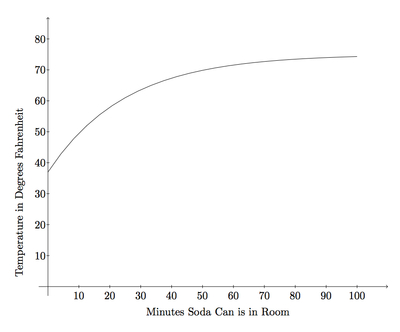# A Saturating Exponential

Alignments to Content Standards: F-LE.B.5 F-BF.B

Suppose a can of cold soda is left in a warm room on a summer day. The graph below shows the temperature of the soda as it gradually increased:The function that describes the temperature, $F$, of the soda (in degrees Fahrenheit) after $t$ minutes can be expressed by $$F(t)=C - R e^{-kt},$$ for some positive values of $C$, $R$, and $k$.<

1. Use the graph to estimate $C$.
2. Use the graph to estimate $R$.
3. What was the approximate room temperature? What was the initial temperature of the soda when placed in the room?

## IM Commentary

The context here is a familiar one: a cold beverage warms once it is taken out of the refrigerator. Rather than giving the explicit function governing this warmth, a graph is presented along with the general form of the function. Students must then interpret the graph in order to understand more specific details regarding the function. In order to complete the task successfully, students must understand the behavior of $e^{-kt}$ as $t$ grows and must also recognize that the complicated expression for $F$ simplifies greatly when $t = 0$ (part (a)).

This task provides an opportunity for students to engage in MP7: having graphed exponential functions before, they should be able to identify the impact of the numbers $R$ and $k$ on the shape of the graph. They will also engage in MP2 as success working on this problem requires relating abstract knowledge about the form of exponential functions with the specific context which this problem models. Also relevant throughout is MP6 as the graph only allows us to approximate the quantities $C$ and $R$ so it is important that students report these quantities accordingly.

This task complements ''Newton's law of cooling.'' In this case the beverage is colder than room temperature and warms up as time passes while for Newton's law, the beverage is warmer than room temperature and cools as time passes.

## Solution

1. To better understand the different constants in the definition of the function $F$ we rewrite the expression for $F$ as $$F(t) = C - \frac{R}{e^{kt}}.$$ Note that since $k$ is positive, the quantity $kt$ grows as $t$ increases. The exponential function $e^{kt}$ grows rapidly and so $\frac{R}{e^{kt}}$ will decrease rapidly. This means that $C$ is the value that $F(t)$ will approach as $t$ grows. Looking at the graph, the temperature of the soda exceeds 70 and appears to be leveling off somewhere between 70 and 80. So the value of $C$ appears to be somewhere between 70 and 80. The values of $F$ are increasing and appear to be in the mid 70's after 100 minutes so $C$ is probably in the mid 70's but could be closer to 80.
2. To find the value of $R$, note that when $t = 0$, $e^{kt} = e^0 = 1$. So $$F(0) = C - R.$$ From part (a), we know that $C$ is between 70 and 80. We can also see from the graph that $F(0)$ is a little less than 40, perhaps 37 or 38. Using this information we see that $R$ lies between 30 and 40, probably closer to 40 since $F(0)$ is definitely less than 40 and $C$ appears to be at least halfway between 70 and 80.
3. The room temperature will be the temperature the soda approaches the longer it stays in the room. So in the equation $C$ is the room temperature and we saw, in part (a), that this appears to be in the mid or upper 70's. The initial temperature of the soda is $F(0)$ which we estimated to be about 37 or 38 in part (b).